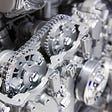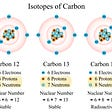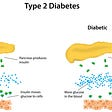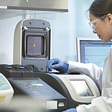# Estimating the Properties of Water

I found a on the by about estimating macroscopic properties of materials using just a few microscopic parameters. I decided to try a version of this analysis myself. It’s based on Lucas’s work, with a few modifications. I focus exclusively on because of its importance for , and make calculations like those and I discuss in the first section of

My goal is to estimate the properties of water using three numbers: the size, mass, and energy associated with water molecules. We take the size to be the center-to-center distance between molecules, which is about 3 , or 3 × 10 ^−10 . The mass of a water molecule is 18 (the ) times the , or about 3 × 10^−26 . The associated with one between water molecules is about 0.2 , or 3 × 10^−20 . This is roughly eight times the thermal energy kT at body temperature, where k is (1.4 × 10^−23 J ^−1) and T is the (310 K). A water molecule has about four hydrogen bonds with neighboring molecules.

## Density

Estimating the of water, ρ, is Homework Problem 4 in Chapter 1 of . Density is mass divided by volume, and volume is distance cubed

ρ = (3 × 10^−26 kg)/(3 × 10^−10 m)3 = 1100 kg m^−3 = 1.1 g cm^−3.

The accepted value is ρ = 1.0 g cm −3, so our calculation is about 10% off; not bad for an order-of-magnitude estimate.

## Compressibility

The of water, κ, is a measure of how the volume of water decreases with increasing pressure. It has dimensions of inverse pressure. The pressure is typically thought of as force per unit area, but we can multiply numerator and denominator by distance and express it as energy per unit volume. Therefore, the compressibility is approximately distance cubed over the total energy of the four hydrogen bonds

κ = (3 × 10^−10 m)3/[4(3 × 10^−20 J)] = 0.25 × 10^−9 −1 = 0.25 GPa^−1 ,

implying a , B (the reciprocal of the compressibility), of 4 GPa. Water has a bulk modulus of about B = 2.2 GPa, so our estimate is within a factor of two.

## Speed of Sound

Once you know the density and compressibility, you can calculate the , c, as (see Eq. 13.11 in IPMB)

c = (ρ κ)^−1/2 = 1/√[(1100 kg m^−3) (0.25 × 10^−9 ^−1)] = 1900 m s^−1 = 1.9 km s^−1.

The measured value of the speed of sound in water is about c = 1.5 km s^−1, which is pretty close for a back-of-the-envelope estimate.

## Latent Heat

A homework problem about vapor pressure in Chapter 3 of IPMB uses water’s , L, which is the energy required to boil water per kilogram. We estimate it as

L = 4(3 × 10^−20 J)/(3 × 10^−26 kg) = 4.0 × 10^6 J kg^−1 = 4 MJ kg^−1.

The known value is L = 2.5 MJ kg^−1. Not great, but not bad.

## Surface Tension

The , γ, is typically expressed as force per unit length, which is equivalent to the energy per unit area. At a surface, we estimate one of the four hydrogen bonds is missing, so

γ = (3 × 10^−20 J)/(3 × 10^−10 m)2 = 0.33 J m^−2 .

The measured value is γ = 0.07 J m^−2, which is about five times less than our calculation. This is a bigger discrepancy than I’d like for an order-of-magnitude estimate, but it’s not horrible.

## Viscosity

The coefficient of , η, has units of kg m −1s −1. We can use the mass of the water molecule in kilograms, and the distance between molecules in meters, but we don’t have a time scale. However, energy has units of kg m 2s ^−2, so we can take the square root of mass times distance squared over energy and get a unit of time, τ

τ = √[(3 × 10^−26 kg) (3 × 10^−10 m)2/4(3 × 10^−20 J)] = 0.15 × 10^−12 s = 0.15 .

We can think of this as a time characterizing the vibrations about equilibrium of the molecules.

The viscosity of water should therefore be on the order of

η = (3 × 10^−26 kg)/[(3 × 10^−10 m) (0.15 × 10^−12 s)] = 0.67 × 10^−3 kg m^−1 s^−1.

Water has a viscosity coefficient of about η = 1 × 10^−3kg m^−1s^−1. I admit this analysis provides little insight into the mechanism underlying viscous effects, and it doesn’t explain the , but it gets the right order of magnitude.

## Specific Heat

The is the energy needed to raise the temperature of water by one degree. implies that the heat capacity is typically equal to Boltzmann’s constant times the per molecule times the number of molecules. The number of degrees of freedom is a subtle thermodynamic concept, but we can approximate it as the number of hydrogen bonds per molecule; about four. Often heat capacity is expressed as the , C, which is the heat capacity per unit mass. In that case, the specific heat is

C = 4 (1.4 × 10^−23 J K^−1)/(3 × 10^−26 kg) = 1900 J K^−1 kg^−1.

The measured value is C = 4200 J K^−1kg^−1, which is more than a factor of two larger than our estimate. I’m not sure why our value is so low, but probably there are degrees of freedom in addition to the four modes we counted.

## Diffusion

The self- constant of water can be estimated using the relating diffusion and viscosity, D = kT/(6π ηa), where a is the size of the molecule. The thermal energy kT is about one eighth of the energy of a hydrogen bond. Therefore,

D = [(3 × 10^−20 J)/8]/[(6)(3.14)(0.67 × 10^−3 kg m^−1 s^−1)(3 × 10^−10 m)] = 10^−9 m^2 s^−1.

Figure 4.11 in IPMB suggests the measured diffusion constant is about twice this estimate: D = 2 × 10^−9 m^2 s^−1.

We didn’t do too bad. Three microscopic parameters, plus the temperature, gave us estimates of density, compressibility, speed of sound, latent heat, surface tension, viscosity, specific heat, and the diffusion constant. This is almost all the properties of water discussed in ’s wonderful book . .

Originally published at .

--

--

--

Professor of Physics at Oakland University and coauthor of the textbook Intermediate Physics for Medicine and Biology.

Love podcasts or audiobooks? Learn on the go with our new app.

## “Metal Complexes” Science-Research, March 2022, Week 4 — summary from NASA Technology Transfer…## “Isotopes ” Science-Research, March 2022 — summary from PubMed, Springer Nature, Wiley Online…## “Obesity and Cancer” Research Papers, September 2021 — summary from Europe PMC## How to Read a Scientific Paper## Of genes and culture, human nature and suffering## Turkey Inspired Technology: A Thanksgiving Startup Tale## “Type 2 Diabetes” Science-Research, January 2022, Week 3 — summary from MedlinePlus Genetics, NCBI…## Four of the Latest Lab Equipment & Technology TrendsProfessor of Physics at Oakland University and coauthor of the textbook Intermediate Physics for Medicine and Biology.

## Differences between American and British English## What’s next for Data Privacy and Stewardship?## Are Electromagnetic Fields Making Me Ill? How Electricity and Magnetism Affect Our Health